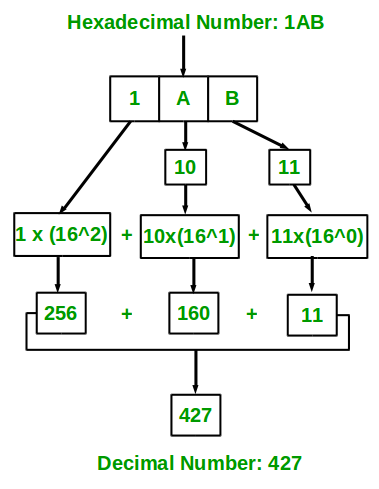Related Articles

# Program for Hexadecimal to Decimal

• Difficulty Level : Easy
• Last Updated : 19 May, 2021

Given a hexadecimal number as input, we need to write a program to convert the given hexadecimal number into an equivalent decimal number.

Examples:

```Input : 67
Output: 103

Input : 512
Output: 1298

Input : 123
Output: 291```

We know that hexadecimal number uses 16 symbols {0, 1, 2, 4, 5, 6, 7, 8, 9, A, B, C, D, E, F} to represent all numbers. Here, (A, B, C, D, E, F) represents (10, 11, 12, 13, 14, 15).

The idea is to extract the digits of a given hexadecimal number starting from the rightmost digit and keep a variable dec_value. At the time of extracting digits from the hexadecimal number, multiply the digit with the proper base (Power of 16) and add it to the variable dec_value. In the end, the variable dec_value will store the required decimal number.

For Example: If the hexadecimal number is 1A.
dec_value = 1*(16^1) + 10*(16^0) = 26

The below diagram explains how to convert a hexadecimal number (1AB) to an equivalent decimal value:Below is the implementation of the above idea.

## Java

 `// Java program to convert hexadecimal to decimal``import` `java.io.*;` `class` `GFG {``    ``// Function to convert hexadecimal to decimal``    ``static` `int` `hexadecimalToDecimal(String hexVal)``    ``{``        ``int` `len = hexVal.length();` `        ``// Initializing base value to 1, i.e 16^0``        ``int` `base = ``1``;` `        ``int` `dec_val = ``0``;` `        ``// Extracting characters as digits from last``        ``// character``        ``for` `(``int` `i = len - ``1``; i >= ``0``; i--) {``            ``// if character lies in '0'-'9', converting``            ``// it to integral 0-9 by subtracting 48 from``            ``// ASCII value``            ``if` `(hexVal.charAt(i) >= ``'0'``                ``&& hexVal.charAt(i) <= ``'9'``) {``                ``dec_val += (hexVal.charAt(i) - ``48``) * base;` `                ``// incrementing base by power``                ``base = base * ``16``;``            ``}` `            ``// if character lies in 'A'-'F' , converting``            ``// it to integral 10 - 15 by subtracting 55``            ``// from ASCII value``            ``else` `if` `(hexVal.charAt(i) >= ``'A'``                     ``&& hexVal.charAt(i) <= ``'F'``) {``                ``dec_val += (hexVal.charAt(i) - ``55``) * base;` `                ``// incrementing base by power``                ``base = base * ``16``;``            ``}``        ``}``        ``return` `dec_val;``    ``}` `    ``// driver program``    ``public` `static` `void` `main(String[] args)``    ``{``        ``String hexNum = ``"1A"``;``        ``System.out.println(hexadecimalToDecimal(hexNum));``    ``}``}`

## Python3

 `# Python3 program to convert``# hexadecimal to decimal` `# Function to convert hexadecimal``# to decimal`  `def` `hexadecimalToDecimal(hexval):` `    ``# Finding length``    ``length ``=` `len``(hexval)` `    ``# Initialize base value to 1,``    ``# i.e. 16*0``    ``base ``=` `1``    ``dec_val ``=` `0` `    ``# Extracting characters as digits``    ``# from last character``    ``for` `i ``in` `range``(length ``-` `1``, ``-``1``, ``-``1``):` `        ``# If character lies in '0'-'9',``        ``# converting it to integral 0-9``        ``# by subtracting 48 from ASCII value``        ``if` `hexval[i] >``=` `'0'` `and` `hexval[i] <``=` `'9'``:``            ``dec_val ``+``=` `(``ord``(hexval[i]) ``-` `48``) ``*` `base` `            ``# Incrementing base by power``            ``base ``=` `base ``*` `16` `        ``# If character lies in 'A'-'F',converting``        ``# it to integral 10-15 by subtracting 55``        ``# from ASCII value``        ``elif` `hexval[i] >``=` `'A'` `and` `hexval[i] <``=` `'F'``:``            ``dec_val ``+``=` `(``ord``(hexval[i]) ``-` `55``) ``*` `base` `            ``# Incrementing base by power``            ``base ``=` `base ``*` `16` `    ``return` `dec_val`  `# Driver code``if` `__name__ ``=``=` `'__main__'``:` `    ``hexnum ``=` `'1A'` `    ``print``(hexadecimalToDecimal(hexnum))` `# This code is contributed by virusbuddah_`

## C#

 `// C# program to convert``// hexadecimal to decimal``using` `System;` `class` `GFG {``    ``// Function to convert``    ``// hexadecimal to decimal``    ``static` `int` `hexadecimalToDecimal(String hexVal)``    ``{``        ``int` `len = hexVal.Length;` `        ``// Initializing base1 value``        ``// to 1, i.e 16^0``        ``int` `base1 = 1;` `        ``int` `dec_val = 0;` `        ``// Extracting characters as``        ``// digits from last character``        ``for` `(``int` `i = len - 1; i >= 0; i--) {``            ``// if character lies in '0'-'9',``            ``// converting it to integral 0-9``            ``// by subtracting 48 from ASCII value``            ``if` `(hexVal[i] >= ``'0'` `&& hexVal[i] <= ``'9'``) {``                ``dec_val += (hexVal[i] - 48) * base1;` `                ``// incrementing base1 by power``                ``base1 = base1 * 16;``            ``}` `            ``// if character lies in 'A'-'F' ,``            ``// converting it to integral``            ``// 10 - 15 by subtracting 55``            ``// from ASCII value``            ``else` `if` `(hexVal[i] >= ``'A'` `&& hexVal[i] <= ``'F'``) {``                ``dec_val += (hexVal[i] - 55) * base1;` `                ``// incrementing base1 by power``                ``base1 = base1 * 16;``            ``}``        ``}``        ``return` `dec_val;``    ``}` `    ``// Driver Code``    ``static` `void` `Main()``    ``{``        ``String hexNum = ``"1A"``;``        ``Console.WriteLine(hexadecimalToDecimal(hexNum));``    ``}``}` `// This code is contributed by mits`

## PHP

 `= 0; ``\$i``--)``    ``{``        ``// if character lies in '0'-'9',``        ``// converting it to integral 0-9``        ``// by subtracting 48 from ASCII value.``        ``if` `(``\$hexVal``[``\$i``] >= ``'0'` `&&``            ``\$hexVal``[``\$i``] <= ``'9'``)``        ``{``            ``\$dec_val` `+= (ord(``\$hexVal``[``\$i``]) - 48) *``                                         ``\$base``;``                ` `            ``// incrementing base by power``            ``\$base` `= ``\$base` `* 16;``        ``}` `        ``// if character lies in 'A'-'F' , ``        ``// converting it to integral``        ``// 10 - 15 by subtracting 55``        ``// from ASCII value``        ``else` `if` `(``\$hexVal``[``\$i``] >= ``'A'` `&&``                 ``\$hexVal``[``\$i``] <= ``'F'``)``        ``{``            ``\$dec_val` `+= (ord(``\$hexVal``[``\$i``]) - 55) *``                                         ``\$base``;``        ` `            ``// incrementing base by power``            ``\$base` `= ``\$base` `* 16;``        ``}``    ``}``    ` `    ``return` `\$dec_val``;``}` `// Driver Code``\$hexNum` `= ``"1A"``;``echo` `hexadecimalToDecimal(``\$hexNum``);` `// This code is contributed by mits``?>`

## Javascript

 ``
Output
`26`

Using predefined function

## Java

 `// Java program to convert hexadecimal to decimal` `import` `java.io.*;` `class` `GFG {``    ``public` `static` `int` `HexToDec(String n)``    ``{``        ``return` `Integer.parseInt(n, ``16``);``    ``}``    ``public` `static` `void` `main(String[] args)``    ``{``        ``String n = ``"1A"``;``        ``System.out.println(HexToDec(n));``    ``}``}`

## Python3

 `# Python program to convert hexadecimal to decimal``def` `HexToDec(n):``    ``return` `int``(n, ``16``);` `if` `__name__ ``=``=` `'__main__'``:``    ``n ``=` `"1A"``;``    ``print``(HexToDec(n));``    ` `# This code is contributed by 29AjayKumar`

## C#

 `// C# program to convert hexadecimal to decimal` `using` `System;` `public` `class` `GFG {``    ``public` `static` `int` `HexToDec(String n)``    ``{``        ``return` `Convert.ToInt32(n, 16);``    ``}``    ``public` `static` `void` `Main(String[] args)``    ``{``        ``String n = ``"1A"``;``        ``Console.WriteLine(HexToDec(n));``    ``}``}` `// This code is contributed by Amit Katiyar`

## Javascript

 ``
Output
`26`

This article is contributed by Harsh Agarwal. If you like GeeksforGeeks and would like to contribute, you can also write an article using write.geeksforgeeks.org or mail your article to contribute@geeksforgeeks.org. See your article appearing on the GeeksforGeeks main page and help other Geeks.